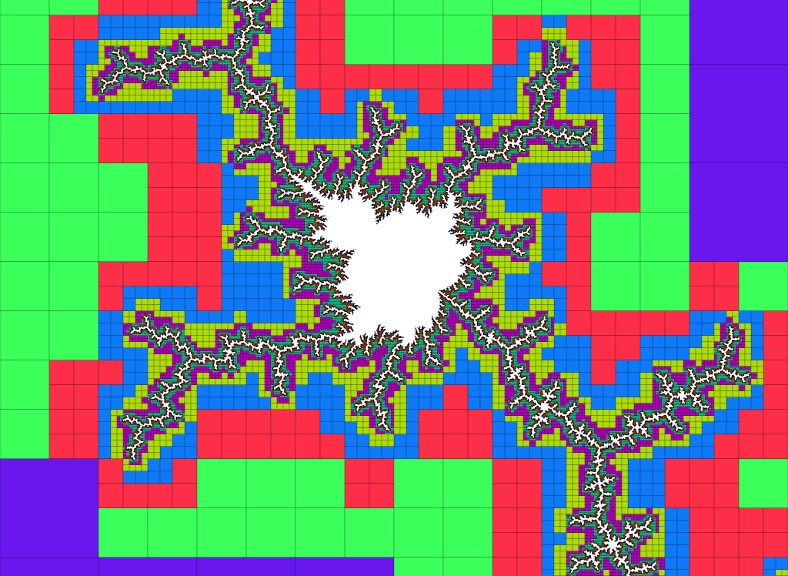# mathr / blog / #

## Distance estimationThis started from a conversation in #haskell, in particular these comments from quicksilver:

quite a lot of the old divide-and-conquer mandelbrot programs from the early 90s used a clever rectangle thing which they could use to fill in large areas of colour fast. is it a mandelbrot theorem that if a rectangle which does not contain either axis has the same iteration number on its entire boundary then the iteration number is constant inside?

I don't know if that holds, but I wrote a short Haskell program that computes exterior distance estimates at the corners of a square, so using some information from Wikipedia:

Once b [the exterior distance estimate for c] is found, by the Koebe 1/4-theorem, we know there's no point of the Mandelbrot set with distance from c smaller than b/4.

it's possible to tell if no point inside that square can possibly be inside the Mandelbrot set. benmachine suggested in #haskell that the method I used to check this is actually not suboptimal, the method being to check if the squares inside the circles-of-exclusion centered on the vertices of the original square do cover all the edges of that square; I have yet to delve into geometry to see if that is correct or if there is an even easier way than this:

exterior :: Square -> Bool
exterior s@(Square a b c d) = fromMaybe False $do lb <- distance a lt <- distance b rb <- distance c rt <- distance d let k = 4 * sqrt 2 * size s return$ and
[ lb + rb > k
, lt + rt > k
, lb + lt > k
, rb + rt > k
]

The code outputs SVG via dirty string concatenation, the above image took 1m15s to render.